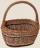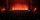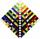# Workman - shift

The worker produces 300 components per shift. How many components would be produced in 18 shift, if his performance gradually increased every shift by 3 components?

Result

x =  5859

#### Solution:Leave us a comment of example and its solution (i.e. if it is still somewhat unclear...):

Showing 0 comments:Be the first to comment!## Next similar examples:

1. Apples in basketsDetermine how many apples are in baskets when in the first basket are 4 apples, and in any other is 29 apples more than the previous, and we have eight baskets.
2. SeatsSeats in the sport hall are organized so that each subsequent row has five more seats. First has 10 seats. How many seats are: a) in the eighth row b) in the eighteenth row
3. StudentsIn the front row sitting three students and in every other row 11 students more than the previous row. Determine how many students are in the room when the room is 9 lines, and determine how many students are in the seventh row.
4. SeriesYour task is express the sum of the following arithmetic series for n = 14: S(n) = 11 + 13 + 15 + 17 + ... + 2n+9 + 2n+11
5. SequenceBetween numbers 1 and 53 insert n members of the arithmetic sequence that its sum is 702.
6. SequenceWrite the first 6 members of these sequence: a1 = 5 a2 = 7 an+2 = an+1 +2 an
7. TreesA certain species of tree grows an average of 0.5 cm per week. Write an equation for the sequence that represents the weekly height of this tree in centimeters if the measurements begin when the tree is 200 centimeters tall.
8. Theorem proveWe want to prove the sentense: If the natural number n is divisible by six, then n is divisible by three. From what assumption we started?
9. Nineteenth memberFind the nineteenth member of the arithmetic sequence: a1=33 d=5 find a19
10. Sequence 3Write the first 5 members of an arithmetic sequence: a4=-35, a11=-105.
11. 6 termsFind the first six terms of the sequence. a1 = 7, an = an-1 + 6
12. Sum of membersWhat is the sum of the first two members of the aritmetic progression if d = -4.3 and a3 = 7.5?
13. Consecutive numbersSum of ten consecutive numbers is 105. Determine these numbers (write first and last).
14. AP - simpleDetermine the first nine elements of sequence if a10 = -1 and d = 4
15. Sequence 2Write the first 5 members of an arithmetic sequence a11=-14, d=-1
16. SequenceWrite the first 7 members of an arithmetic sequence: a1=-3, d=6.
17. AS sequenceIn an arithmetic sequence is given the difference d = -3 and a71 = 455. a) Determine the value of a62 b) Determine the sum of 71 members.